# Clicker Heroes

 93 7 43M•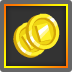complete
•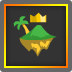complete
•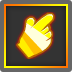complete
•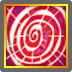complete
•complete
•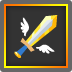complete
•complete
 Plays 42,741,716 Ratings 35,650 Favorites 38,446 Published Sep 22, 2014

## Description

Kill monsters, collect gold, upgrade heroes, use skills, find treasure, kill bosses, and explore new worlds in this epic adventure!

## Controls

Click on the monsters.

Legend of Large Numbers
1K = 1,000 = One Thousand
1M = 1,000K = One Million
1B = 1,000M = One Billion
1T = 1,000B = One Trillion
1q = 1,000T = One Quadrillion
1Q = 1,000q = One Quintillion
1s = 1,000Q = One Sextillion
1S = 1,000s = One Septillion
1O = 1,000S = One Octillion
1N = 1,000O = One Nonillion
1d = 1,000N = One Decillion
1U = 1,000d = One Undecillion
1D = 1,000U = One Duodecillion
1! = 1,000D = One Tredecillion
1@ = 1,000! = One Quattuordecillion
1# = 1,000@ = One Quindecillion
1\$ = 1,000# = One Sexdecillion
1% = 1,000\$ = One Septendecillion
1^ = 1,000% = One Octodecillion
1& = 1,000^ = One Novemdecillion
1* = 1,000& = One Vigintillion
A lot > 1,000* < A lot

## May 20, 2020

Hey Heroes! Introducing Clans 2.0 with Patch 1.0e12! Battle the revamped Immortals for more rubies every day! The old clan system is still available as well and has been renamed Legacy Raids.

## March 9, 2018